Tamilnadu State Board New Syllabus Samacheer Kalvi 8th Maths Guide Pdf Chapter 1 Numbers Ex 1.7 Text Book Back Questions and Answers, Notes.

## Tamilnadu Samacheer Kalvi 8th Maths Solutions Chapter 1 Numbers Ex 1.7

Miscellaneous Practice Problems

Question 1.
If $$\frac{3}{4}$$ of a box of apples weighs 3kg and 225 gm, how much does a full box of apples weigh?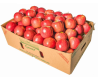Let the total weight of a box of apple = x kg.
Weight of $$\frac{3}{4}$$ of a box apples = 3 kg 225 gm.
= 3.225kg
$$\frac{3}{4}$$ × x = 3225
x = $$\frac{3.225 \times 4}{3}$$ kg
= 1.075 × 4kg = 4.3kg
= 4 kg 300 gm
Weight of the box of apples = 4 kg 300 gm.Question 2.
Mangalam buys a water jug of capacity 3$$\frac{4}{5}$$ litre. If she buys another jug which is 2$$\frac{2}{3}$$ times as large as the smaller jug, how many litre can the larger one hold?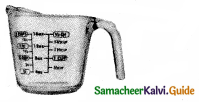Capacity of the small waterug = 3$$\frac{4}{5}$$ litres.
Capacity of the big jug = $$2 \frac{2}{3}$$ times the small one.
= $$2 \frac{2}{3} \times 3 \frac{4}{5}=\frac{8}{3} \times \frac{19}{5}=\frac{152}{15}$$
= $$\frac{2}{15}$$ litres
Capacity of the large jug = $$\frac{2}{15}$$ litres.

Question 3.
Ravi multiplied $$\frac { 25 }{ 8 }$$ and $$\frac { 16 }{ 5 }$$ to obtain $$\frac { 400 }{ 120 }$$. He says that the simplest form of this product is $$\frac { 10 }{ 3 }$$ and Chandru says the answer in the simplest form is $$3 \frac{1}{3}$$. Who is correct? (or) Are they both correct? Explain.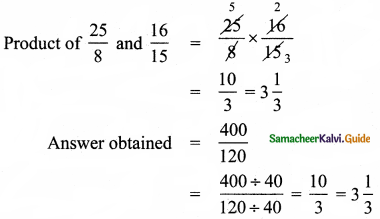∴ The product is $$\frac{400}{120}$$ and its simplest form improper fraction is $$\frac{10}{3}$$
And mixed fraction is $$3 \frac{1}{3}$$
∴ Both are correctQuestion 4.
Find the length of a room whose area is $$\frac{153}{10}$$ sq.m and whose breadth is $$2 \frac{11}{20}$$m.
Length of the room × Breadth = Area of the room
Breadth of the room = $$2 \frac{11}{20}$$ m
Area of the room = $$\frac{153}{10}$$ sq.m
Length x $$2\frac{11}{20}$$ = $$\frac{153}{10}$$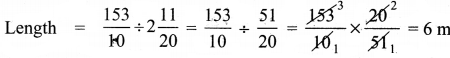Length of the room = 6 m

Question 5.
There is a large square portrait of a leader that covers an area of 4489 cm2. 1f each side has a 2 cm liner, what would be its area?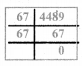Area of the square = 4489 cm2
(side)2 = 4489 cm2
(side)2 = 67 × 67
side = 672
Length of a side = 67
Length of a side with liner = 67 + 2 + 2 cm
= 71 cm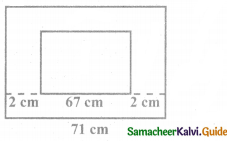Area of the larger square = 71 × 71 cm2
= 5041 cm2
Area of the liner = Area of big square – Area of small square
= (5041 – 4489) cm2
= 552 cm2Question 6.
A greeting card has an area 90 cm2. Between what two whole numbers is the length of its side?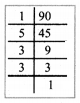Area of the greeting card = 90 cm2
(side)2 = 90 cm2
(side)2 = 2 × 5 × 3 × 3 = 2 × 5 × 32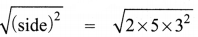Side = 3$$\sqrt{2 \times 5}$$
side = 3√10 cm
side = 3 × 3.2cm
side = 9.6 cm
∴ Side lies between the whole numbers 9 and 10.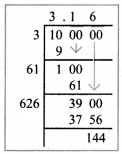Question 7.
225 square shaped mosaic tiles, each of area 1 square decimetre exactly cover a square shaped verandah. How long is each side of the square shaped verandah?
Area of one tile = 1 sq.decimeter
Area of 225 tiles = 225 sq.decimeter
225 square tiles exactly covers the square shaped verandah.
∴ Area of 225 tiles = Area of the verandah
Area of the verandah = 225 sq.decimeter
side × side = 15 × 15 sq.decimeter
side = 15 decimeters
Length of each side of verandah = 15 decimeters.Question 8.
If $$\sqrt{1906624} \times \sqrt{x}$$ = 31oo, find x.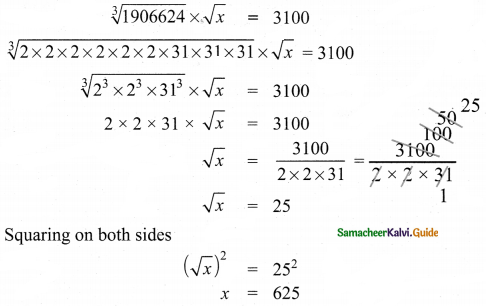Question 9.
If 2m – 1 + 2m + 1 = 640, then find ‘m’.
Given 2m – 1 + 2m + 1 = 640
2m – 1 + 2m + 1 = 128 + 512
2m – 1 + 2m + 1 – 27 + 29
m – 1 = 7
m = 7 + 1
m = 8
[consecutive powers of 2]

Powers of 2:
2, 4, 8, 16, 32, 64, 128, 256, 512,….Question 10.
Give the answer in scientific notation:
A human heart beats at an average of 80 beats per minute. How many times does it beat in
i) an hour?
ii) a day?
iii) a year?
iv) 100 years?
Heart beat per minute = 80 beats
(i) an hour
One hour = 60 minutes
Heart beat in an hour = 60 × 80
= 4800
= 4.8 × 103

(ii) In a day
One day = 24 hours = 24 × 60 minutes
∴ Heart beat in one day = 24 × 60 × 80 = 24 × 4800 = 115200
= 1.152 × 105

(iii) a year
One year = 365 days = 365 × 24 hours = 365 × 24 × 60 minutes
∴ Heart beats in a year = 365 × 24 × 60 × 80
= 42048000
= 4.2048 × 107(iv) 100 years
Heart beats in one year = 4.2048 × 107
heart beats in 100 years = 4.2048 × 107 × 100 = 4.2048 × 107 × 102
= 4.2048 × 109

Challenging Problems:

Question 11.
In a map, if 1 inch refers to 120 km, then find the distance between two cities B and C which are $$4\frac{1}{6}$$ inches and $$3\frac{1}{3}$$ inches from the city A which lies between the cities B and C.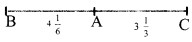1 inch = 120 km
Distance between A and B = $$4\frac{1}{6}$$
Distance between A and C = $$3\frac{1}{3}$$
∴ Distance between B and C = $$4 \frac{1}{6}+3 \frac{1}{3}$$ inches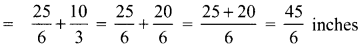1 inch = 120km
∴ $$\frac{45}{6}$$ inches = $$\frac{45}{6}$$ × 120 km = 900 km
Distance between B and C = 900 kmQuestion 12.
Give an example and verify each of the following statements.
(i) The collection of all non-zero rational numbers is closed under division.
let a = $$\frac{5}{6}$$ and b = $$\frac{-4}{3}$$ be two non zero rational numbers.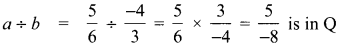∴ Collection of non-zero rational numbers are closed under division.

(ii) Subtraction is not commutative for rational numbers.
let a = $$\frac{1}{2}$$ and b = $$-\frac{5}{6}$$ be two rational numbers.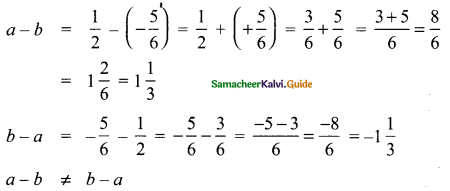a – b ≠ b – a
∴ Subtraction is not commutative for rational numbers.(iii) Division is not associative for rational numbers.
Let a = $$\frac{2}{5}$$, b = $$\frac{6}{5}$$, c = $$\frac{3}{5}$$ be three rational numbers.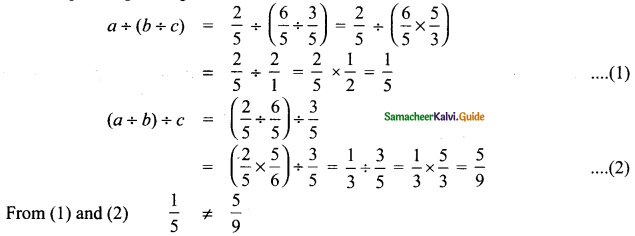a ÷ (b ÷ c) ≠ (a ÷ b) ÷ c
∴ Division is not associative for rational numbers.

(iv) Distributive property of multiplication over subtraction is true for rational numbers. That is, a (b – c) = ab – ac.
Let a = $$\frac{2}{9}$$, b = $$\frac{3}{6}$$, c = $$\frac{1}{3}$$ be three rational numbers.
To prove a × (b – c) = ab – bc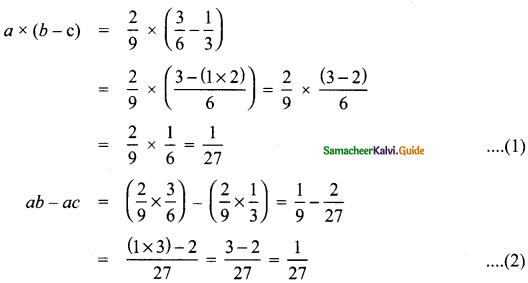∴ From (1) and (2)
a × (b – c) = ab – bc
∴ Distributivity of multiplication over subtraction is true for rational numbers.(v) The mean of two rational numbers is rational and lies between them.
Let a = $$\frac{2}{11}$$ and b = $$\frac{5}{6}$$ be two rational numbers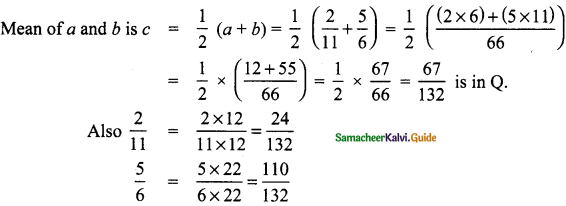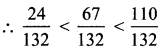∴ The mean lies between the given rational numbers $$\frac{2}{11}$$ and $$\frac{5}{6}$$

Question 13.
If $$\frac { 1 }{ 4 }$$ of a ragi adai weighs 120 grams, what will be the weight of $$\frac { 2 }{ 3 }$$ of the same ragi adai ?
Let the weight of 1 ragi adai = x grams
given $$\frac { 1 }{ 4 }$$ of x = 120gm
$$\frac { 1 }{ 4 }$$ × x = 120
x = 120 × 4
x = 480gm
∴ $$\frac { 2 }{ 3 }$$ of the adai = $$\frac { 2 }{ 3 }$$ × 480 gm = 2 × 160 gm = 320gm
$$\frac { 2 }{ 3 }$$ of the weight of adai = 320gmQuestion 14.
If p + 2q =18 and pq = 40, find $$\frac{2}{p}+\frac{1}{q}$$
Given p + 2q = 18 ……… (1)
pq = 40 ……… (2)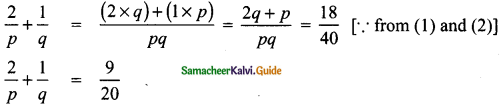Question 15.
Find x if $$5 \frac{x}{5} \times 3 \frac{3}{4}$$ = 21.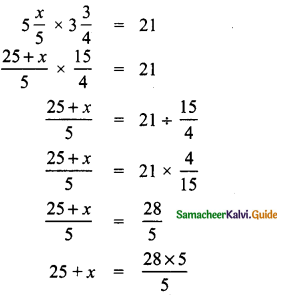25 + x = 28
x = 28 – 25
x = 3Question 16.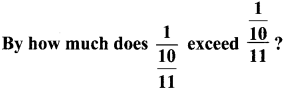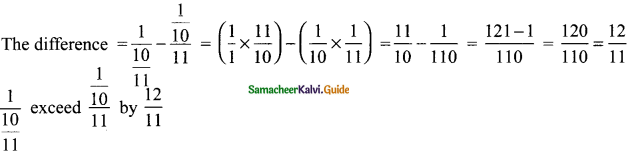Question 17.
A group of 1536 cadets wanted to have a parade forming a square design. Is it possible? If it is not possible, how many more cadets would be required?
Number of cadets to form square design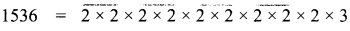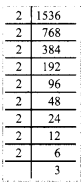The numbers 2 and 3 are unpaired
∴ It is impossible to have the parade forming square design with 1536 cadets.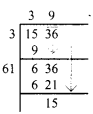39 × 39 = 1521
Also 40 × 40 = 1600
∴ We have to add (1600 – 1536) = 64 to make 1536 a perfect square.
∴ 64 more cadets would be required to form the square design.Question 18.
Evaluate: $$\sqrt{286225}$$ and use it to compute $$\sqrt{2862.25}+\sqrt{28.6225}$$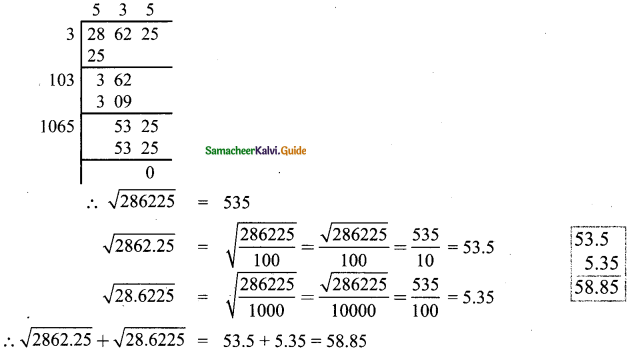Question 19.
Simplify: (3.769 × 105) + (4.21 × 105)
(3.769 × 105) + (4.21 × 105) = 3,76,900 + 4,21,000
= 7,97,000
= 7.979 × 105Question 20.
Order the following from the least to the greatest: 1625, 8100, 3500, 4400, 2600